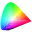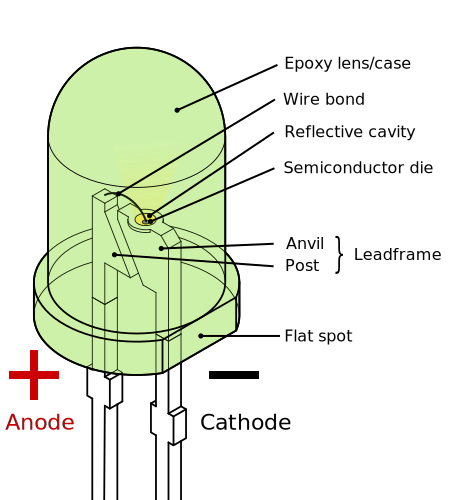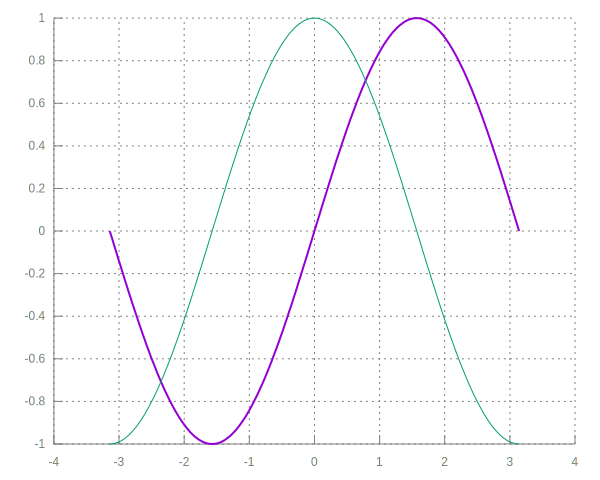# IPerl display demo¶

author: Zaki Mughal

date: 2015-02-09

This notebook demonstrates how to use the rich display system in IPerl and how it can be extended on the fly.

All data that is displayed implements a Displayable role. This role requires a method called iperl_data_representations that returns a HashRef of different representations of the data where the keys are the MIME type of the data (e.g., text/html) and values are the strings that contain the bytestream for that MIME type. For example, with PNG, we have

In :
use v5.16;
use DDP; # Data::Printer

my $png_display = Devel::IPerl::Display::PNG->new( "http://www.libpng.org/pub/png/PngSuite/ccwn3p08.png" ); say &np( [ keys %{$png_display->iperl_data_representations } ] );

$png_display;  [  "image/png",  "text/html",  "text/plain" ]Notice that I just displayed that PNG by just putting the $png_display variable at the end? That's because any displayable is automatically displayed if it is at the end of a cell.

But there's a problem: I don't want to type or remember Devel::IPerl::Display::PNG every time I want to load up a PNG.

Instead, you can just call the helper method IPerl->png() and you'll get the same result.

In :
IPerl->png( "http://www.libpng.org/pub/png/PngSuite/ccwn3p08.png" );There are other Displayables too. For example, let's load up an <iframe>.

In :
my $iframe_display = IPerl->iframe( "http://metacpan.org/recent", width => "75%" );  What if we want to display multiple things in one cell? For example, we want to loop over a number of images and display each of them? You can do that by calling the IPerl->display() on the Displayable object. In : my @svg = split ' ', q[ https://upload.wikimedia.org/wikipedia/commons/f/fd/Ghostscript_Tiger.svg https://upload.wikimedia.org/wikipedia/commons/f/f9/LED,_5mm,_green_(en).svg ]; IPerl->display( IPerl->svg($_ , width => "200" ) ) for @svg;

$png_display;There is also a way to display arbitrary HTML. Here, we create a simple HTML table that loops over a 2D nested ArrayRef. We could send the string directly to the IPerl->html() method, but that isn't very DRY. Instead, we'll create our own helper! In : IPerl->helper( my_table => sub { my ($self, $data) = @_; return unless ref$data eq 'ARRAY';

my $html = "<table>"; for my$row (@$data) {$html .= "<tr>";
for my $cell (@$row) {
my $cell_html =$cell->iperl_data_representations->{"text/html"};
$html .= "<td>$cell_html</td>\n";
}
$html .= "</tr>"; }$html .= "</table>";

IPerl->html( $html ); }); my$N = 4; my $M = 10; my$d = [ ([ ( $png_display ) x$M ]) x $N ]; IPerl->my_table($d  );There are other plugins besides the Displayables that work directly on file types (PNG, SVG, HTML, etc.).

For example, you can load a plugin that adds a role to PDL::Graphics::Gnuplot and makes it displayable as an SVG.

In :
IPerl->load_plugin( "PDLGraphicsGnuplot" );
use PDL;
use PDL::Graphics::Gnuplot;
use PDL::Constants qw(PI);

my $gp = gpwin(); # do some styling$gp->option( topcmd => <<'GP');
# define axis
# remove border on top and right and set color to gray
set style line 11 lc rgb '#808080' lt 1
set border 3 back ls 11
set tics nomirror

# define grid
set style line 12 lc rgb '#808080' lt 0 lw 1
set grid back ls 12
GP

my $theta = zeros(200)->xlinvals(-1*PI, 1*PI);$gp->plot( { lw => 2 }, $theta, sin($theta), {}, $theta, cos($theta)  );

IPerl->display( IPerl->tex( q| $\sin\theta$ and $\cos\theta$ | ) );

$gp; $\sin\theta$and$\cos\theta\$WARNING - dumping ON - gnuplot commands go to the terminal only.
at /home/zaki/perl5/perlbrew/perls/perl-5.26.1/lib/site_perl/5.26.1/Devel/IPerl/Plugin/PDLGraphicsGnuplot.pm line 40.

(killed gnuplot)
at /home/zaki/perl5/perlbrew/perls/perl-5.26.1/lib/site_perl/5.26.1/Devel/IPerl/Plugin/PDLGraphicsGnuplot.pm line 56.


Have fun and let me know what you make with your IPerl notebooks!